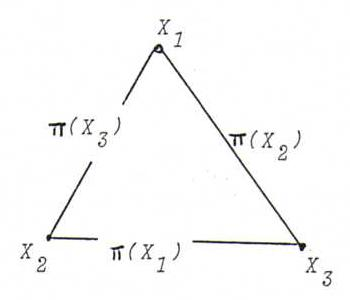### COURSE OF PROJECTIVE GEOMETRY

§ 19:

O75: There are no autopolar points or lines. Indeed, a straight line in ℜ3 can't be perpendicular to a plane and lie in that plane at the same time.

O76: Conditions : X1 and X2 not autopolar, X1 and X2 conjugate. Then for X3 := π(X1).π(X2) we have π(X3) = X1X2.O77: (Dualise the proofs.)
i) The intersection point of two autopolar lines can't be autopolar.
ii) A polarity induces on each pencil of lines L where L isn't autopolar an involution whose pairs are conjugate lines (or identity).

O78: Let l be a line with at least three autopolar points. Then l isn't autopolar (first proposition §19), so we have the projectivity θ: X → π(X).l.
The fixed points of θ are exactly the autopolar points of π on l. Since there are at least three such points, θ is identity (FT).